# Introduction to Algorithms 6 046 J18 401 JSMA

• Slides: 29
Download presentationIntroduction to Algorithms 6. 046 J/18. 401 J/SMA 5503 Lecture 10 Prof. Erik Demaine © 2001 by Charles E. Leiserson Introduction to Algorithms 1Balanced search trees Balanced search tree: A search-tree data structure for which a height of O(lg n) is guaranteed when implementing a dynamic set of n items. • AVL trees • 2 -3 -4 trees Examples: • B-trees • Red-black trees © 2001 by Charles E. Leiserson Introduction to Algorithms 2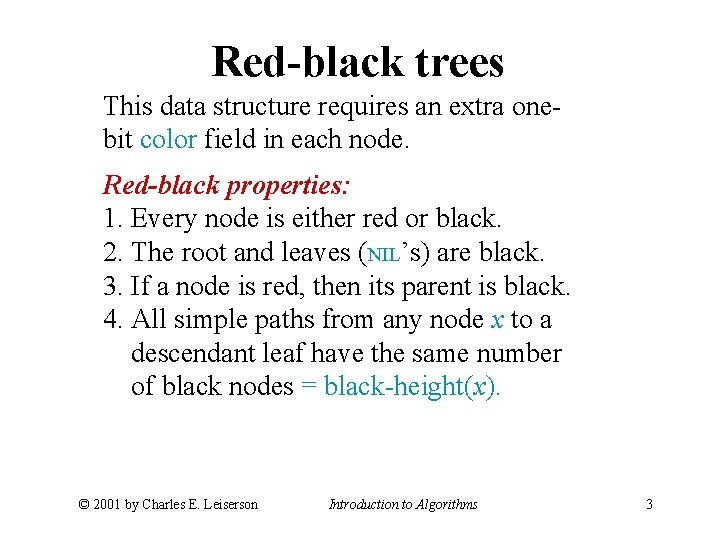Red-black trees This data structure requires an extra onebit color field in each node. Red-black properties: 1. Every node is either red or black. 2. The root and leaves (NIL’s) are black. 3. If a node is red, then its parent is black. 4. All simple paths from any node x to a descendant leaf have the same number of black nodes = black-height(x). © 2001 by Charles E. Leiserson Introduction to Algorithms 3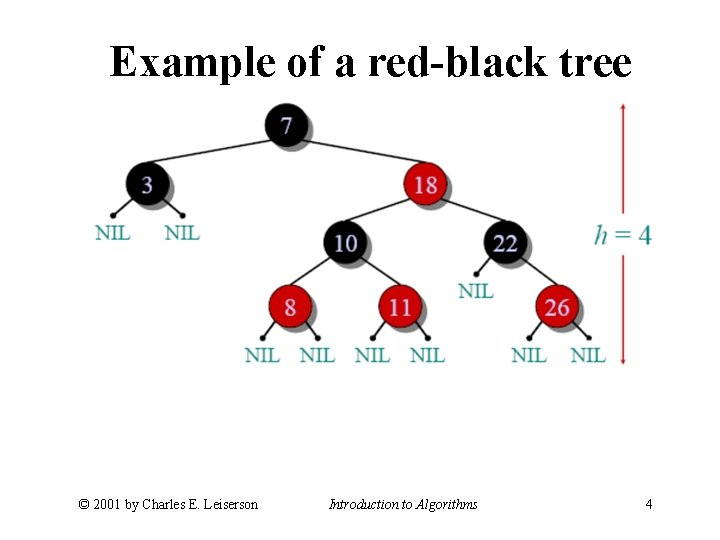Example of a red-black tree © 2001 by Charles E. Leiserson Introduction to Algorithms 4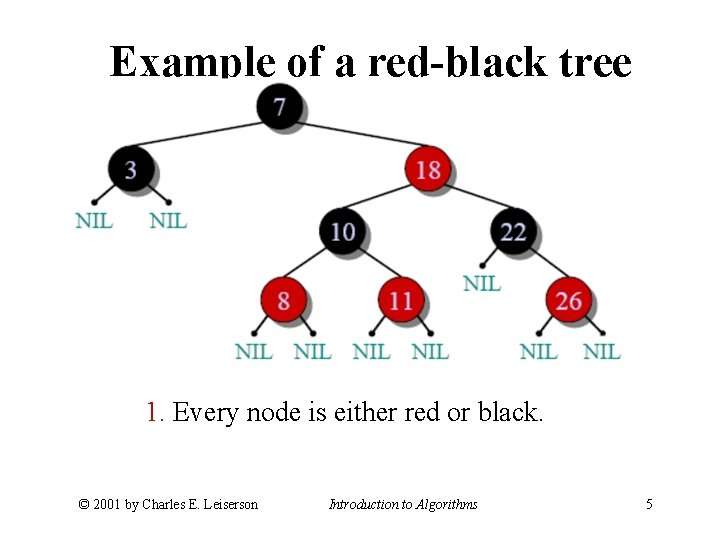Example of a red-black tree 1. Every node is either red or black. © 2001 by Charles E. Leiserson Introduction to Algorithms 5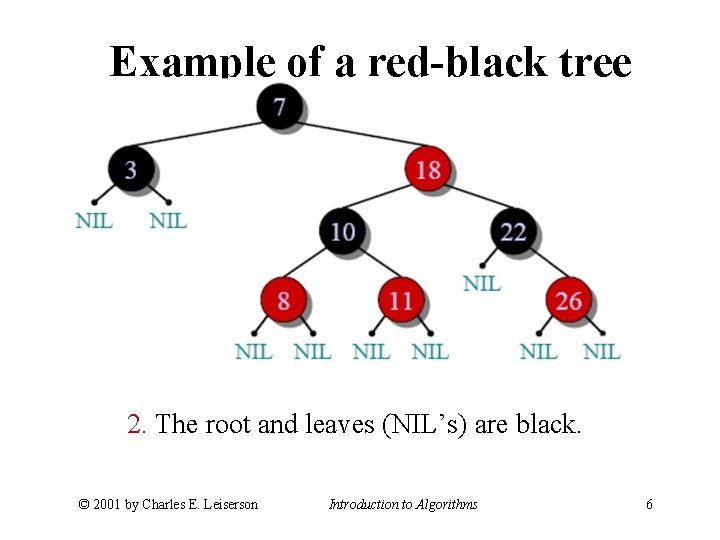Example of a red-black tree 2. The root and leaves (NIL’s) are black. © 2001 by Charles E. Leiserson Introduction to Algorithms 6Example of a red-black tree 3. If a node is red, then its parent is black. © 2001 by Charles E. Leiserson Introduction to Algorithms 7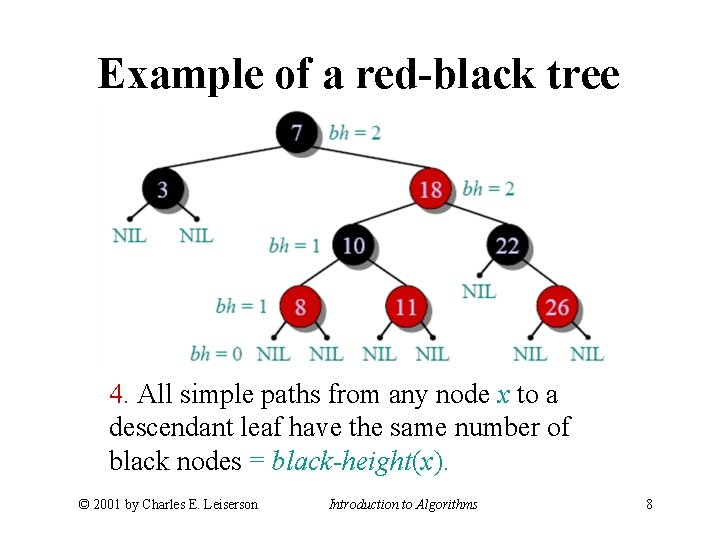Example of a red-black tree 4. All simple paths from any node x to a descendant leaf have the same number of black nodes = black-height(x). © 2001 by Charles E. Leiserson Introduction to Algorithms 8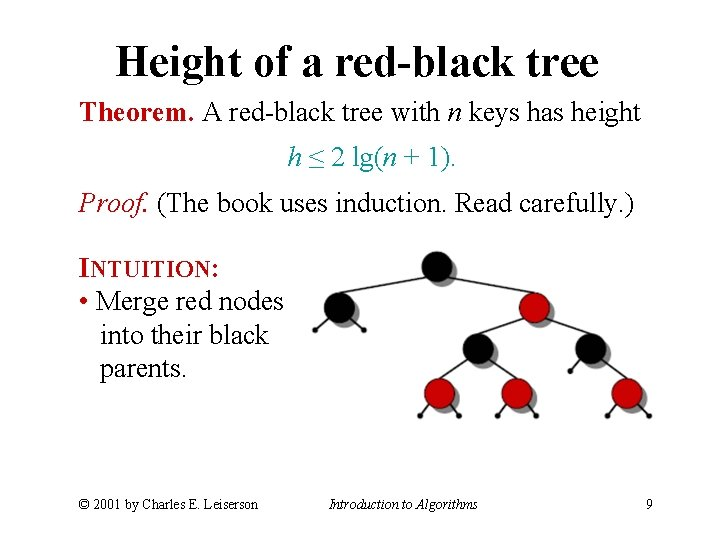Height of a red-black tree Theorem. A red-black tree with n keys has height h ≤ 2 lg(n + 1). Proof. (The book uses induction. Read carefully. ) INTUITION: • Merge red nodes into their black parents. © 2001 by Charles E. Leiserson Introduction to Algorithms 9Height of a red-black tree Theorem. A red-black tree with n keys has height h ≤ 2 lg(n + 1). Proof. (The book uses induction. Read carefully. ) INTUITION: • Merge red nodes into their black parents. © 2001 by Charles E. Leiserson Introduction to Algorithms 10Height of a red-black tree Theorem. A red-black tree with n keys has height h ≤ 2 lg(n + 1). Proof. (The book uses induction. Read carefully. ) INTUITION: • Merge red nodes into their black parents. © 2001 by Charles E. Leiserson Introduction to Algorithms 11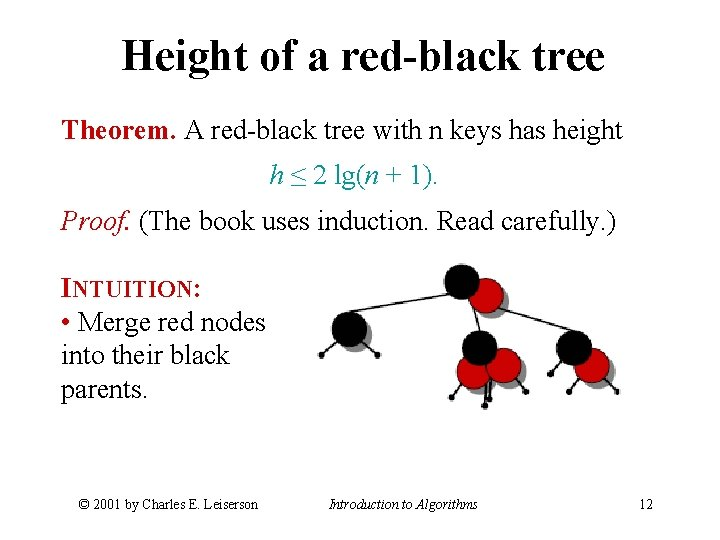Height of a red-black tree Theorem. A red-black tree with n keys has height h ≤ 2 lg(n + 1). Proof. (The book uses induction. Read carefully. ) INTUITION: • Merge red nodes into their black parents. © 2001 by Charles E. Leiserson Introduction to Algorithms 12Height of a red-black tree Theorem. A red-black tree with n keys has height h ≤ 2 lg(n + 1). Proof. (The book uses induction. Read carefully. ) INTUITION: • Merge red nodes into their black parents. © 2001 by Charles E. Leiserson Introduction to Algorithms 13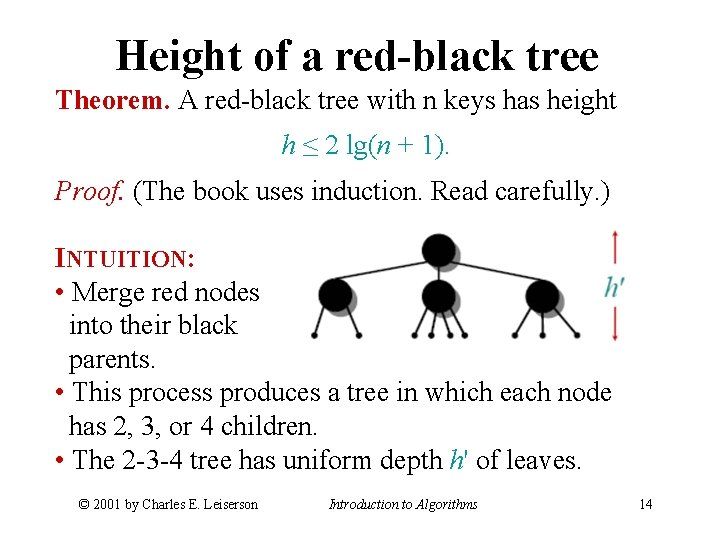Height of a red-black tree Theorem. A red-black tree with n keys has height h ≤ 2 lg(n + 1). Proof. (The book uses induction. Read carefully. ) INTUITION: • Merge red nodes into their black parents. • This process produces a tree in which each node has 2, 3, or 4 children. • The 2 -3 -4 tree has uniform depth h' of leaves. © 2001 by Charles E. Leiserson Introduction to Algorithms 14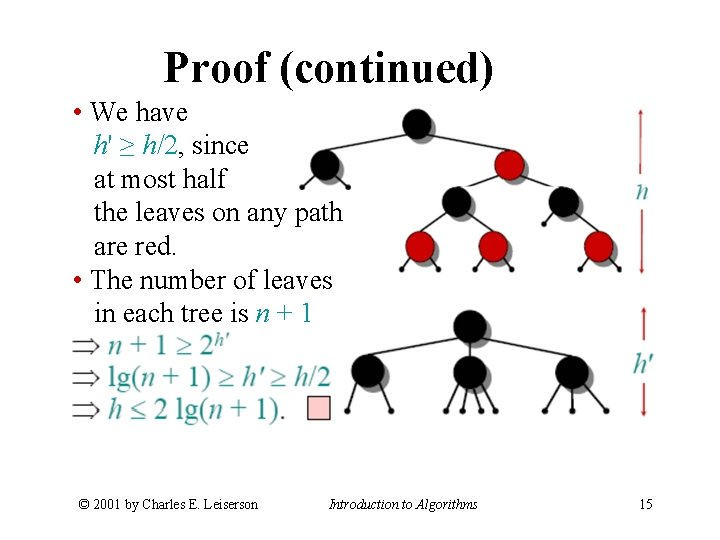Proof (continued) • We have h' ≥ h/2, since at most half the leaves on any path are red. • The number of leaves in each tree is n + 1 © 2001 by Charles E. Leiserson Introduction to Algorithms 15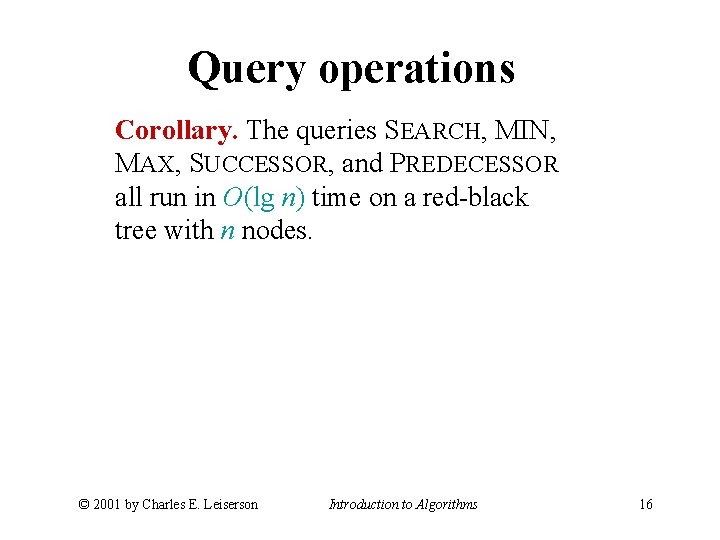Query operations Corollary. The queries SEARCH, MIN, MAX, SUCCESSOR, and PREDECESSOR all run in O(lg n) time on a red-black tree with n nodes. © 2001 by Charles E. Leiserson Introduction to Algorithms 16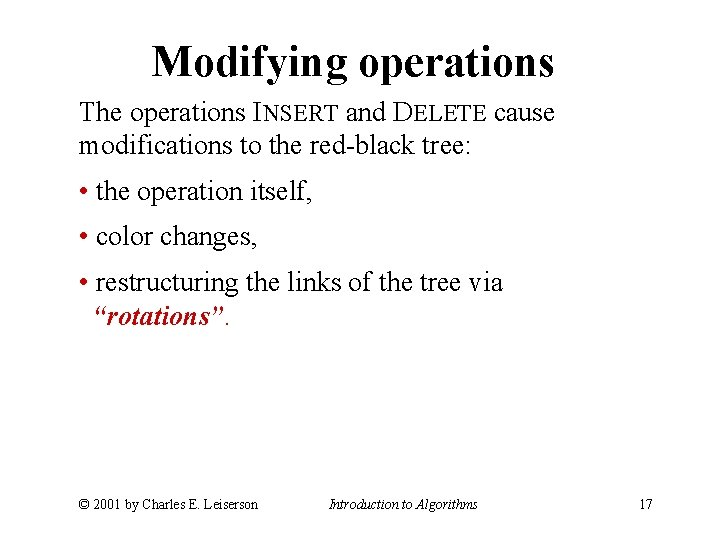Modifying operations The operations INSERT and DELETE cause modifications to the red-black tree: • the operation itself, • color changes, • restructuring the links of the tree via “rotations”. © 2001 by Charles E. Leiserson Introduction to Algorithms 17Rotations maintain the inordering of keys: • a ∈ α, b ∈ β, c ∈ γ ⇒ a ≤ A ≤ b ≤ B ≤ c. A rotation can be performed in O(1) time. © 2001 by Charles E. Leiserson Introduction to Algorithms 18Insertion into a red-black tree IDEA: Insert x in tree. Color x red. Only redblack property 3 might be violated. Move the violation up the tree by recoloring until it can be fixed with rotations and recoloring. Example: © 2001 by Charles E. Leiserson Introduction to Algorithms 19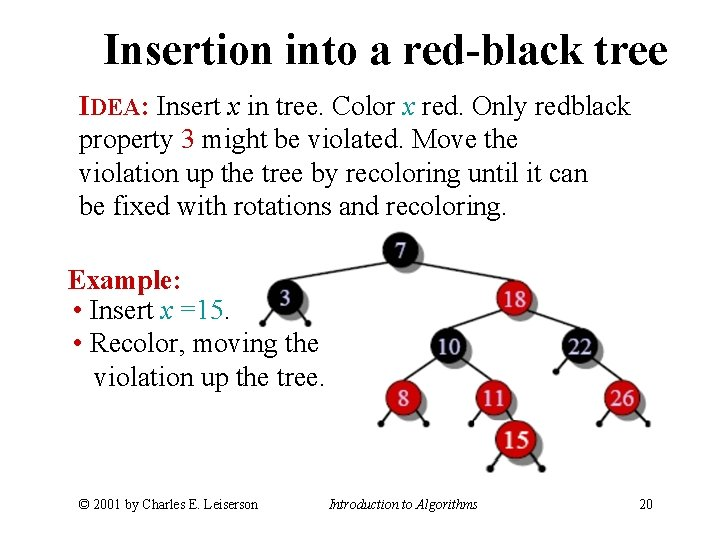Insertion into a red-black tree IDEA: Insert x in tree. Color x red. Only redblack property 3 might be violated. Move the violation up the tree by recoloring until it can be fixed with rotations and recoloring. Example: • Insert x =15. • Recolor, moving the violation up the tree. © 2001 by Charles E. Leiserson Introduction to Algorithms 20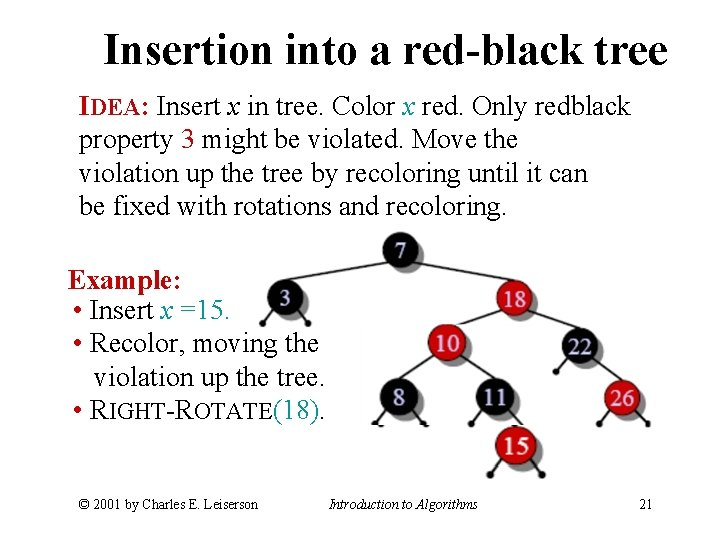Insertion into a red-black tree IDEA: Insert x in tree. Color x red. Only redblack property 3 might be violated. Move the violation up the tree by recoloring until it can be fixed with rotations and recoloring. Example: • Insert x =15. • Recolor, moving the violation up the tree. • RIGHT-ROTATE(18). © 2001 by Charles E. Leiserson Introduction to Algorithms 21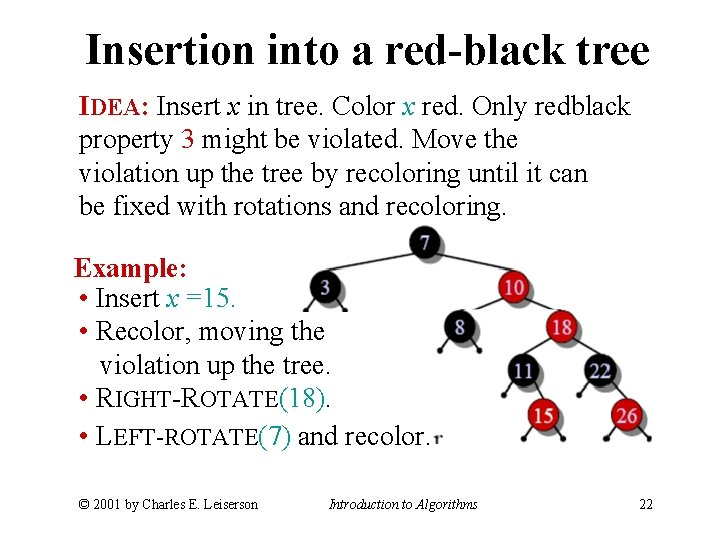Insertion into a red-black tree IDEA: Insert x in tree. Color x red. Only redblack property 3 might be violated. Move the violation up the tree by recoloring until it can be fixed with rotations and recoloring. Example: • Insert x =15. • Recolor, moving the violation up the tree. • RIGHT-ROTATE(18). • LEFT-ROTATE(7) and recolor. © 2001 by Charles E. Leiserson Introduction to Algorithms 22Insertion into a red-black tree IDEA: Insert x in tree. Color x red. Only redblack property 3 might be violated. Move the violation up the tree by recoloring until it can be fixed with rotations and recoloring. Example: • Insert x =15. • Recolor, moving the violation up the tree. • RIGHT-ROTATE(18). • LEFT-ROTATE(7) and recolor. © 2001 by Charles E. Leiserson Introduction to Algorithms 23Pseudocode RB-INSERT(T, x) TREE-INSERT(T, x) color[x] ← RED ⊳ only RB property 3 can be violated while x ≠ root[T] and color[p[x]] = RED do if p[x] = left[p[p[x]] then y ← right[p[p[x]] ⊳ y = aunt/uncle of x if color[y] = RED then �Case 1� else if x = right[p[x]] then �Case 2�⊳ Case 2 falls into Case 3 �Case 3� else �“then” clause with “left” and “right” swapped� © 2001 by Charles E. Leiserson Introduction to Algorithms 24 color[root[T]] ← BLACKGraphical notation Let denote a subtree with a black root. All s have the same black-height. © 2001 by Charles E. Leiserson Introduction to Algorithms 25Case 1 (Or, children of A are swapped. ) © 2001 by Charles E. Leiserson Push C’s black onto A and D, and recurse, since C’s parent may be red. Introduction to Algorithms 26Case 2 Transform to Case 3. © 2001 by Charles E. Leiserson Introduction to Algorithms 27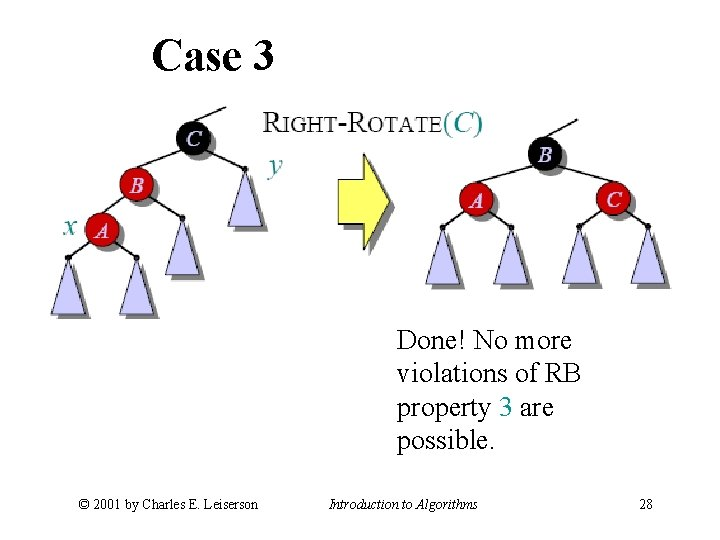Case 3 Done! No more violations of RB property 3 are possible. © 2001 by Charles E. Leiserson Introduction to Algorithms 28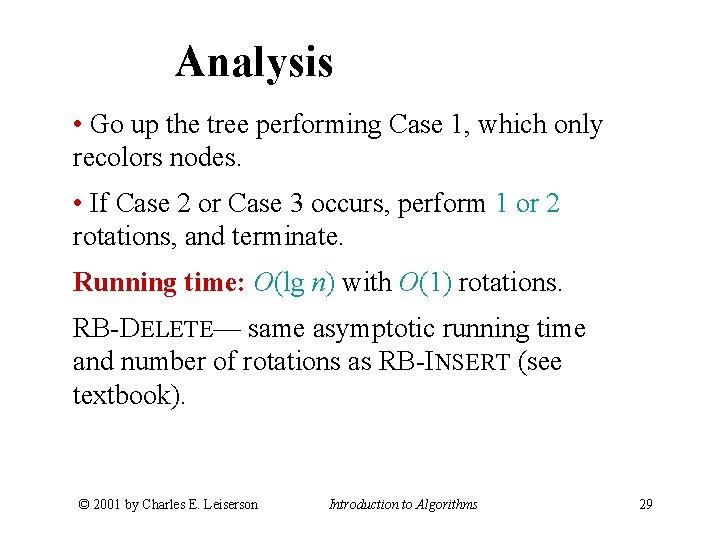Analysis • Go up the tree performing Case 1, which only recolors nodes. • If Case 2 or Case 3 occurs, perform 1 or 2 rotations, and terminate. Running time: O(lg n) with O(1) rotations. RB-DELETE— same asymptotic running time and number of rotations as RB-INSERT (see textbook). © 2001 by Charles E. Leiserson Introduction to Algorithms 29